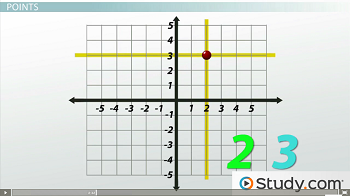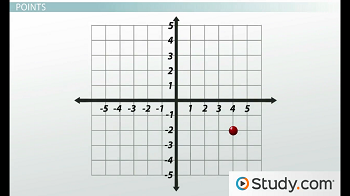# The Cartesian Coordinate System: Plotting Points & Graphing Lines

An error occurred trying to load this video.

Try refreshing the page, or contact customer support.

Coming up next: How to Use The Midpoint Formula

### You're on a roll. Keep up the good work!

Replay
Your next lesson will play in 10 seconds
• 0:01 Cartesian Coordinate System
• 1:56 Points
• 3:13 Plotting Points
• 4:11 Graphing Lines
• 8:11 Lesson Summary

Want to watch this again later?

Timeline
Autoplay
Autoplay
Speed

#### Recommended Lessons and Courses for You

Lesson Transcript
Instructor: Yuanxin (Amy) Yang Alcocer

Amy has a master's degree in secondary education and has taught math at a public charter high school.

Watch this video lesson to see how useful the Cartesian coordinate system is. Learn how points are labeled and how you can plot points and graph lines using the system.

## The Cartesian Coordinate System

The Cartesian coordinate system is a system that tells you your exact position on a graph. Why is this useful? It is useful because you can use this system to graph a series of points that will provide solutions to your equations. The equations that are used with this system will have two variables, one for each axis.

The Cartesian coordinate system uses a horizontal axis that is called the x-axis and a vertical axis called the y-axis. Equations for lines in this system will have both the x and y variable. For example, the equation 2x + y = 2 is an example of a line in this system. Lots of equations can be written with these two variables. You can have equations that explain population growth and you can have equations that explain your cell phone bill.

What does a Cartesian coordinate system look like? It looks like graph paper with a dark line for the x-axis and another dark line for the y-axis. These two axes cross perpendicular to each other. The point where they cross is at point 0 for each axis. This graph extends forever in all directions. Both axes are labeled with numbers according to the number line.

You can think of the x-axis as a horizontal number line and the y-axis as a vertical number line. When you draw one, though, you only draw it as big as you need it to be. If you only need the x-axis and the y-axis to go up to 10, then you can stop there for both axes. Let's look at some examples to see how it works. Let's start with points.

## Points

Before plotting points, let's talk about how points are labeled. Points are labeled based on their position on the graph in relation to both the x-axis and the y-axis. For example, if you look at this point and you draw an imaginary vertical line straight down, you see that it lands where the x-axis equals 2. This x-axis number will be the first part of your label.Drawing an imaginary horizontal line, you see that it crosses the y-axis where it equals 3. This is the second part of the label for that point. To label this point, then, you write the first part and second part separated by a comma inside a pair of parentheses like this: (2, 3).

Look at this second point. Try labeling this one on your own. Where does the imaginary vertical line cross the x-axis? What about the imaginary horizontal line?Because the imaginary vertical line crosses the x-axis at 4 and the imaginary horizontal line crosses the y-axis at -2, the point is labeled (4, -2).

## Plotting Points

Now that you know how points are labeled, plotting points becomes easier. Plotting points is essentially labeling points in reverse.

For example, if you are given the point (0, 3) to plot, you would first find the vertical imaginary line where the x value is 0 and then you would follow this line either up or down until you reach the horizontal imaginary line where the y value is 3. Once you've found it, place a dot on the graph and label it with (0, 3).

You try plotting the point (-1, 2). You first find the vertical imaginary line where the x-axis is -1. Once you've found it, you follow it up to where the y-axis is 2. You have found the point. Now place a point there and label it with (-1, 2). You did it!

## Graphing Lines

To graph lines, we first calculate two or more points and then we plot them. There are two ways you can graph a line. You can create a table of two or more points or you can calculate the x- and y-intercepts to plot. The intercepts are where the imaginary lines from the point cross each axis.

Let's try graphing the line 2x + y = 2 using both the table method and the intercept method. For the table method, we first create a table with two columns, one for the x-axis and one for the y-axis like this:

x y

We can choose to calculate just two points or more. I recommend calculating three points so you can be sure you have a straight line. To calculate them, you set the x value and then find the y value using algebra.

The easiest numbers to choose for the x value are -1, 0, and 1. I recommend using these. Let's see what happens when we plug in these numbers into our equation of a line. This is what happens when x = -1:

2(-1) + y = 2

-2 + y = 2

-2 + 2 + y = 2 + 2

y = 4

So our first point is (-1, 4). We can fill our table like this:

x y
-1 4

Moving on, this is what happens when x = 0:

2(0) + y = 2

0 + y =2

y = 2

To unlock this lesson you must be a Study.com Member.

### Register to view this lesson

Are you a student or a teacher?

### Unlock Your Education

#### See for yourself why 30 million people use Study.com

##### Become a Study.com member and start learning now.
Back
What teachers are saying about Study.com

### Earning College Credit

Did you know… We have over 160 college courses that prepare you to earn credit by exam that is accepted by over 1,500 colleges and universities. You can test out of the first two years of college and save thousands off your degree. Anyone can earn credit-by-exam regardless of age or education level.